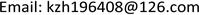﻿ 积分因子存在性的充要条件及其应用 The Necessary and Sufficient Condition for the Existence of Integrating Factors with Its Application

Pure Mathematics
Vol.08 No.03(2018), Article ID:24826,6 pages
10.12677/PM.2018.83027

The Necessary and Sufficient Condition for the Existence of Integrating Factors with Its Application

Zhihong Kong

Department of Mathematics, Taiyuan Normal University, Jinzhong ShanxiReceived: Apr. 20th, 2018; accepted: May 4th, 2018; published: May 11th, 2018ABSTRACT

In this paper, we give the necessary and sufficient condition for the existence of integrating factors and their proof. Meanwhile, some examples are given for its application.

Keywords:Integrating Factors, Exact First-Order Differential Equations, Arbitrary Differentiable Functions, Integrating Factors for Grouping1. 引言

$M\left(x,y\right)\text{d}x+N\left(x,y\right)\text{d}y=0$ (1)

2. 定理的证明

$\frac{\partial u}{\partial x}\text{d}x+\text{d}y\frac{\partial u}{\partial y}=0$ (2)

$\frac{\text{d}y}{\text{d}x}=-\frac{\partial u}{\partial x}/\frac{\partial u}{\partial y}.$ (3)

$\frac{\text{d}y}{\text{d}x}=-\frac{M}{N}$ (4)

$\frac{\partial u}{\partial x}/\frac{\partial u}{\partial y}=\frac{M}{N},$

$\frac{\partial u}{\partial x}/M=\frac{\partial u}{\partial y}/N.$

$\frac{\partial u}{\partial x}=\mu M$ , $\frac{\partial u}{\partial y}=\mu N.$

$\mu \left(x,y\right)$ 乘以(1)两端，得

$\mu M\text{d}x+\mu N\text{d}y=0,$

$\frac{\partial u}{\partial x}\text{d}x+\frac{\partial u}{\partial y}\text{d}x=0,$

$\begin{array}{c}\frac{\partial }{\partial y}\left[\mu \phi \left(u\right)M\right]=\phi \left(u\right)\frac{\partial \left(uM\right)}{\partial y}+\mu M{\phi }^{\prime }\left(u\right)\frac{\partial u}{\partial y}\\ =\phi \left(u\right)\frac{\partial \left(uM\right)}{\partial y}+\mu M{\phi }^{\prime }\left(u\right)\mu N\\ =\phi \left(u\right)\frac{\partial \left(uM\right)}{\partial y}+{\mu }^{2}MN{\phi }^{\prime }\left(u\right)\end{array}$ (5)

$\begin{array}{c}\frac{\partial }{\partial y}\left[\mu \phi \left(u\right)N\right]=\phi \left(u\right)\frac{\partial \left(uN\right)}{\partial x}+\mu N{\phi }^{\prime }\left(u\right)\frac{\partial u}{\partial x}\\ =\phi \left(u\right)\frac{\partial \left(uN\right)}{\partial x}+\mu N{\phi }^{\prime }\left(u\right)\mu M\\ =\phi \left(u\right)\frac{\partial \left(uN\right)}{\partial x}+{\mu }^{2}MN{\phi }^{\prime }\left(u\right)\end{array}$ (6)

$\frac{\partial \left(uM\right)}{\partial y}=\frac{\partial \left(uN\right)}{\partial x},$

$\frac{\partial }{\partial y}\left[\mu \phi \left(u\right)M\right]=\frac{\partial }{\partial x}\left[\mu \phi \left(u\right)N\right].$

$\mu M\text{d}x+\mu N\text{d}y=\text{d}u$$\overline{\mu }M\text{d}x+\overline{\mu }N\text{d}y=\text{d}v,$

$\mu M=\frac{\partial u}{\partial x},\text{\hspace{0.17em}}\mu N=\frac{\partial u}{\partial y},\text{\hspace{0.17em}}\overline{\mu }M=\frac{\partial v}{\partial x},\text{\hspace{0.17em}}\overline{\mu }N=\frac{\partial v}{\partial y}$

$\frac{\partial \left(u,y\right)}{\partial \left(x,y\right)}=|\begin{array}{cc}\frac{\partial u}{\partial x}& \frac{\partial u}{\partial y}\\ \frac{\partial \nu }{\partial x}& \frac{\partial \nu }{\partial y}\end{array}|=|\begin{array}{cc}\mu M& \mu N\\ \overline{\mu }M& \overline{\mu }N\end{array}|\equiv 0$

$\frac{\partial u}{\partial x}\cdot \frac{\partial u}{\partial y}=\mu M\cdot \mu N\overline{)\equiv }0,$

$v\left(x,y\right)=\psi \left(u\left(x,y\right)\right).$

$\overline{\mu }\left(M\text{d}x+N\text{d}y\right)=\text{d}v=\text{d}\psi \left(u\right)={\psi }^{\prime }\left(u\right)\text{d}u={\psi }^{\prime }\left(u\right)\mu \left(M\text{d}x+N\text{d}y\right),$

3. 应用举例

${\mu }_{2}\left(M\text{d}x+N\text{d}y\right)=\text{d}u,$

$u\left(x,y\right)=c$ (任意常数)为方程(1)的通解。根据上述定理知 ${\mu }_{1}={\mu }_{2}\phi \left(u\right)$ ，其中 $\phi \left(t\right)$ 是t的可微函数，于是

$\frac{{\mu }_{1}}{{\mu }_{2}}=\phi \left(u\right).$ (7)

$0\equiv \text{d}\phi \left(u\right)\equiv {\phi }^{\prime }\left(u\right)\text{d}u\equiv {\phi }^{\prime }\left(u\right){\mu }_{2}\left(M\text{d}x+N\text{d}y\right).$

${\mu }_{2}\overline{)\equiv }0$$\phi \left(u\right)$ 不恒为常数，即 ${\phi }^{\prime }\left(u\right)\overline{)\equiv }0$ ，故 $M\text{d}x+N\text{d}y\equiv 0$ ，即对 $\phi \left(u\right)=c$ 微分后推导出 $M\text{d}x+N\text{d}y\equiv 0$ 。这说明由 $\phi \left(u\right)=c$ (任意常数)所确定的y与x的关系式是方程(1)的解，故 $\frac{{\mu }_{1}}{{\mu }_{2}}=c$ (任意常数)是(1)的通解。

$xM\left(x,y\right)+yN\left(x,y\right)=c$

$x\left(4y\text{d}x+2x\text{d}y\right)+{y}^{3}\left(3y\text{d}x+5x\text{d}y\right)=0.$

$x\left(4y\text{d}x+2x\text{d}y\right)=x\left[\left(2y\text{d}x+2x\text{d}y\right)+2x\text{d}y\right]=x\left[2\text{d}\left(xy\right)+2y\text{d}x\right]=2x\text{d}\left(xy\right)+2xy\text{d}x=\text{d}\left(2{x}^{2}y\right)$

${\mu }_{1}=1,\text{\hspace{0.17em}}{u}_{1}=2{x}^{2}y.$

${y}^{3}\left(3y\text{d}x+5x\text{d}y\right)=3{y}^{4}\text{d}x+5x{y}^{3}\text{d}y.$

$\frac{3}{x}\text{d}x+\frac{5}{y}\text{d}y=3\text{d}\left(\mathrm{ln}|x|\right)+5\text{d}\left(\mathrm{ln}|y|\right)=\text{d}\left(\mathrm{ln}|{x}^{3}{y}^{5}|\right),$

$1\cdot \phi \left(2{x}^{2}y\right)=\frac{1}{x{y}^{4}}\stackrel{˜}{\phi }\left(\mathrm{ln}|{x}^{3}{y}^{5}|\right).$

$\phi \left(2{x}^{2}y\right)=2{x}^{2}y$$\stackrel{˜}{\phi }\left(\mathrm{ln}|{x}^{3}{y}^{5}|\right)=2{x}^{3}{y}^{5}$ ，则得到原方程的一个积分因子 $\mu =2{x}^{2}y$ 。用它乘原方程两边，得

$2{x}^{2}y\text{d}\left(2{x}^{2}y\right)+6{x}^{2}{y}^{5}\text{d}x+10{x}^{3}{y}^{4}\text{d}y=0.$

$\frac{1}{2}\text{d}{\left(2{x}^{2}y\right)}^{2}+2{y}^{5}\text{d}{x}^{3}+2{x}^{3}\text{d}{y}^{5}=\text{d}\left(2{x}^{4}{y}^{2}\right)+\text{d}\left(2{x}^{2}{y}^{5}\right)=\text{d}\left(2{x}^{4}{y}^{2}+2{x}^{3}{y}^{5}\right)=0,$

${x}^{4}{y}^{2}+{x}^{3}{y}^{5}=c,$

$\left({x}^{3}y-2{y}^{2}\right)\text{d}x+{x}^{4}\text{d}y=0.$

$\left({x}^{3}y\text{d}x+{x}^{4}\text{d}y\right)+\left(-2{y}^{2}\text{d}x\right)=0,$

${x}^{3}\left(y\text{d}x+x\text{d}y\right)+\left(-2{y}^{2}\text{d}x\right)=0.$ (8)

$\frac{1}{{x}^{3}}\phi \left(xy\right)=\frac{1}{-2{y}^{2}}\stackrel{˜}{\phi }\left(x\right).$

$\phi \left(xy\right)=\frac{1}{{\left(xy\right)}^{2}}$$\stackrel{˜}{\phi }\left(x\right)=-\frac{2}{{x}^{5}}$ ，则得到原方程的一个积分因子

$\mu =\mu \left(x,y\right)=\frac{1}{{x}^{5}{y}^{2}}.$

$\frac{1}{{x}^{2}{y}^{2}}\text{d}\left(xy\right)-\frac{2}{{x}^{5}}\text{d}y=0,$

$\text{d}\left(-\frac{1}{xy}\right)+\text{d}\left(\frac{1}{2{x}^{4}}\right)=0,$

$\text{d}\left(-\frac{1}{xy}+\frac{1}{2{x}^{4}}\right)=0.$

$-\frac{1}{xy}+\frac{1}{2{x}^{4}}=c,$

The Necessary and Sufficient Condition for the Existence of Integrating Factors with Its Application[J]. 理论数学, 2018, 08(03): 215-220. https://doi.org/10.12677/PM.2018.83027

1. 1. 王高雄, 周之铭, 朱思铭, 等. 常微分方程[M]. 第3版. 北京: 高等教育出版社, 2006: 61.

2. 2. 丁同仁, 李承治. 常微分方程[M]. 第2版. 北京: 高等教育出版社, 2004: 49-50.